# Deep stuff about deep learning?

Unless you live a secluded life without internet (in which case you’re not reading those lines), odds are that you have heard and read about deep learning (such as in this 2012 article in the New York Times, or the Wired’s article about the new Facebook AI lab).

So what is deep learning? Well, from my current limited understanding, it seems to be a pretty simple supervised learning technique which can be explained in a few lines. Of course, simple problems can be very deep (ever heard of the Collatz conjecture?), and it looks like this may be the case with deep learning. After explaining what is deep learning (or more accurately what is my understanding of deep learning), I will point out some recent theoretical progress towards understanding the basic phenomenona at play. Let me be clear right away: it seems to me that no one has a real clue about what’s going on.

Deep learning: it’s all about the features

Ok, so, you have a data setwith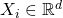and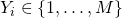. Typicallyis an image, andis say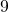if there is a pinguin in this image. A very efficient algorithm to learn a mapping fromtois to use some kind of linear classification, such as multi-class logistic regression (see also this post for some background on linear classification). However applying a linear classification directly ontypically does not work very well, and this why we usually first map the pointto, where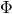is some feature map. Designinghas been part of the art of machine learning, and the idea behind deep learning is to incorporate the problem of finding a good map as part of the learning process (again in the previous post on linear classification I also discussed this possibility in the context of SVM, though we ended up with a semi-definite program which does not scale well with large data sets).

Ok so what kind ofdo we look at in deep learning? Well they are of the following form, for some sequence ofmatrices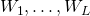, with:The (non-linear) mappingsare fixed and part of the design choice (i.e., they are not learned). The parametersare also specified beforehand. Furthermore one usually impose extra constraints on the matrices, such as having all columns bounded in-norm (say by).

The game now is simply to incorporate the matricesas free variables when one performs the optimization of the multiclass logistic loss. This optimization is always performed with Stochastic Gradient Descent (or a simple variant of it, such as mini-batch SGD, see this post and Section 6.2 in my survey): take an example in your data set; compute the gradient of the logistic loss at this example (with respect to the matricesand the logistic regression weights), and do a small step in the opposite direction of the gradient.

Design choices

Part of the success of deep learning is in the choices left open above, such as the choice of the non-linear maps, and the set of constraints on the matrices. Let me partly describe some of these choices that Krizhevsky, Sutskever and Hinton used for their computer vision breakthrough where they basically halfed the error rate of the competition on the ImageNet dataset (the details can be found here).

First they take. Thenand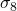are the so-called ReLU function (Rectified Linear Unit), which simply take the positive part coordinate-wise. On the other hand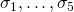are composition of ReLU with max-pooling. The max-pooling is a dimension reduction operation, which simply takesadjacent coordinates (in the case of an image,adjacent pixels) and returns the maximal element. Regarding the constraints on the matrices,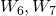andare left unconstrained (besides perhaps the energy control on the columns), whileare highly structure convolutional networks, or ConvNets as they are usually called. Essentially this means that in these matrices, the columnsup to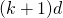are translations of some very sparse vector. Precisely, for, they take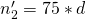and each sparse vector corresponds to aimage. In other words the vector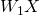corresponds to a collection ofimages, each one of them being the convolution ofwith a smallpatch (whose weights are being learned).

While this post is not about the history of deep learning (this has been discussed at length pretty much everywhere on the internet), I still would like to point out that the apparently arbitrary choice behind max-pooling and the ConvNets are due to Yann LeCun, who has experimented with these for many many years.

Some mysterious steps

So far I would say that the method described above kind of make sense (apart from the max-pooling operation which I really do not understand). But things are now going to turn out a bit more wacky than you might have expected. First note that there is one key element missing from my description of the learning algorithm: what does “small” mean in the sentence “take a small step in the opposite direction of the gradient”. If you know about convex optimization and machine learning, then you know that a lot of work usually goes into understanding precisely what kind of learning rate one should take, and in particular at which rate one should decrease it over time. In deep learning all of this theory goes out of the window since the objective function is not convex (note that there is some convexity in the sense that the objective is convex in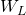– thanks to Nicolas Le Roux for fixing a mistake in the first version of the previous sentence). Here it seems that the current state of the art is to start with some guess, say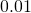, then look at how the training error decays as more examples are being processed, and if the training error plateau then just divide the learning rate by.

Another point which seems key to the success of such an architecture is to control overfitting. Again for a convex problem we know that one-pass through the data with SGD and the appropriate learning rate would work just fine in terms of generalization error. In deep learning the algorithm makes multiple passes on the data, and furthermore the problem is non-convex. This strongly suggests that overfitting will be a real problem, especially given the number of free parameters. Thankfully Hinton and his colleagues invented a quite smart technique, called “dropout”. The idea is simply to take the gradient with respect to only half of the columns of the matrices, where the half is chosen at random at each iteration. In the network view of the architecture this amounts to “dropping” each unit with probability. Intuitively this forces the network to be more robust, and to “repeat” itself, thus avoiding overfitting.

Hacking through life

Of course, if you want to beat everyone else in the world at something, you better have every parts of your system completely optimized. In that respect the deep learning community took the implementation of the above ideas to the next level by using GPUs, thus making the computation of the gradients much faster (by the way, the ReLU non-linearity also helps here, compared to more traditional non-linearities such as sigmoids). Various other tricks are being used, such as artificially expanding the data set by altering the(e.g., by translating the images) or by running the network backward.

Towards an understanding of deep learning?

I think it’s fair to say that we do not understand why deep nets work at all, and we understand even less why they are doing so much better than anything else. The three main questions are: (i) why is SGD meaningful for this non-convex problem, (ii) why is dropout enough to avoid overfitting, (iii) can we explain why ConvNets are a sensible way to learn representations?

Very limited progress has been made on these questions, though I will cite some papers that at least try to attack these problems. I try to summarize each work with one sentence, see the papers for more details (and in particular the details will reveal that these papers do not make direct progress on the above questions).

– Sanjeev Arora and his co-authors proved that a “random and sparse” deep net can be learned in polynomial time.

– Roi Livni, Shai Shalev-Shwartz and Ohad Shamir obtain some results on the approximation power of a neural network with a non-linearity of the form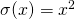.

– Gerard Ben Arous, Yann LeCun and their students draw an interesting connection between fully connected neural networks (such as the last 3 layers in the above architecture) and spherical spin glasses.

– Stephane Mallat gives some insights on (iii) via the scattering transform. Arnab Paul and Suresh Venkat borrow ideas from group theory to shed some light on (iii).

– Francis Bach proves some generalization bounds for neural networks with one hidden layer.

I apologize for misrepresenting deep learning so badly in this post. I know I missed a lot, and you’re welcome to correct me in the comments!

– Approaches for (ii): David McAllester with PAC-Bayesian bounds, and Percy Liang and students with a link to AdaGrad.

– Approach to (iii) by a group at Facebook on the relevance of ConvNets for certain natural distributions.

– I said in the post that all optimization theory goes out of the window when the problem is non-convex. This is of course not true, and for instance a 1998 paper by Leon Bottou has results for SGD in more general scenario. However in the non-convex case we only have very weak guarantees which basically say nothing about the global optimum.

This entry was posted in Machine learning. Bookmark the permalink.

## 19 Responses to "Deep stuff about deep learning?"

••••••••••••••••••